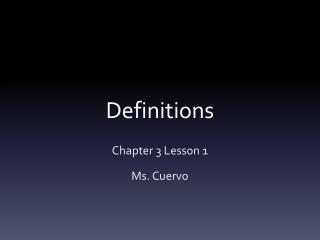DownloadDownload PresentationDefinitions

# Definitions

Download Presentation## Definitions

- - - - - - - - - - - - - - - - - - - - - - - - - - - E N D - - - - - - - - - - - - - - - - - - - - - - - - - - -
##### Presentation Transcript

1. Definitions Chapter 3 Lesson 1 Ms. Cuervo

2. Vocabulary • Parallel Lines-Coplanar lines that do not intersect • Skew Lines-noncoplanar lines. Neither parallel or intersecting. • Parallel Planes-planes that do not intersect A LINE AND A PLANE ARE PARALLEL IF THEY DON’T INTERSECT.

3. Vocabulary • Transversal-a line that intersects two or more coplanar lines in different points. • Alternate Interior Angles-two nonadjacent interior angles on opposite sides of a transversal • Same-side Interior Angles-two interior angles on the same side of a transversal • Corresponding Angles-two angles in corresponding positions relative to the two lines.

4. Theorem 3-1 • If two parallel planes are cut by a third plane, then the lines of intersection are parallel.

5. Properties of Parallel Lines Chapter 3 Lesson 2 Ms. Cuervo

6. Postulates • If two parallel lines are cut by a transversal, then corresponding angles are congruent.

7. Theorems 3-2 • If two parallel lines are cut by a transversal, then the alternate interior angles are congruent.

8. Theorem 3-3 • If two parallel lines are cut by a transversal, then the same-side interior angles are supplementary.

9. Theorem 3-4 • If a transversal is perpendicular to one of two parallel lines, then it is perpendicular to the other one also.

10. Proving Lines Parallel Chapter 3 Lesson 3 Ms. Cuervo

11. Postulates • Postulate 10-If two parallel lines are cut by a transversal, then the corresponding angles are congruent. • Postulate 11-If two lines are cut by a transversal and corresponding angles are congruent, then the lines are parallel.

12. Theorem 3-5 • If two lines are cut by a transversal and alternate interior angles are congruent, then the lines are parallel.

13. Theorem 3-6 • If two lines are cut by a transversal and the same-side interior angles are supplementary, then the lines are parallel.

14. Theorem 3-7 • In a plane two lines perpendicular to the same line are parallel.

15. Theorem 3-8 Through a point outside a line, there is exactly one line parallel to the given line.

16. Theorem 3-9 • Through a point outside a line, there is exactly one line perpendicular to the given line.

17. Theorem 3-10 • Two lines parallel to the third line are parallel to each other.

18. Angles of a Triangle Chapter 3 Lesson 4 Ms. Cuervo

19. Vocabulary • Triangle-a figure formed by three segments joining three noncollinear points. • Vertex-The points when line segments intersect (plural is vertices). • Sides-segments of the triangle

20. Vocabulary • Scalene Triangle-No sides are congruent • Isosceles Triangle-At least two sides congruent • Equilateral Triangle-All sides congruent

21. Vocabulary • Acute Triangle-Three acute angles • Obtuse Triangle-One obtuse angle • Right Triangle-One right angle • Equiangular Triangle-All angles are congruent.

22. Vocabulary • Auxiliary Line-a line (or ray or segment) added to a diagram to help in a proof. • Corollary-a statement that can be proved easily by applying a theorem.

23. Theorem • The sum of the measures of the angles of a triangle is 180.

24. Corollaries • Corollary 1-If two angles of one triangle are congruent to two angles of another triangle, then the third angles are congruent. • Corollary 2-Each angle of an equiangular triangle has the measure of 60.

25. Corollaries • Corollary 3-In a triangle, there can be at most one right angle or obtuse angle. • Corollary-The acute angles of a right triangle are complementary.

26. Theorem 3-12 • The measure of an exterior angle of a triangle equals the sum of the measures of the two remote interior angles.

27. Angles of a Polygon Chapter 3 Lesson 5 Ms. Cuervo

28. Vocabulary • Polygon- “many angles” • Convex Polygon-a polygon such that no line containing a side of the polygon contains a point in the interior of the polygon. • Diagonal-segments joining two nonconsecutive vertices • Regular Polygon-a polygon that is both equiangular and equilateral.

29. Theorem 3-13 • The sum of the measures of the angles of a convex polygon with n sides is (n-2)180 • Each individual angle is n-2(180) n

30. Theorem 3-14 • The sum of the measures of the exterior angles of any convex polygon, one angle at each vertex, is 360.

31. Inductive Reasoning Chapter 3 Lesson 6 Ms. Cuervo

32. Vocabulary • Inductive Reasoning-reasoning that is widely used in science and every day life

33. Example 1 • After picking marigolds for the first time, Connie began to sneeze. She also began sneezing the next four times she was near marigolds. Based on this past experience, Connie reasons inductively that she is allergic to marigolds.

34. Example 2 • Every time Josephhas thrown a high curve ball to Angel, Angel has gotten hit. Josephconcludes from his experience that it is not a good idea to pitch high curve balls to Angel. • In coming to this conclusion, Joseph has used inductive reasoning. It may be that Angeljust happened not to be lucky those times, but Josephis too bright to feed another high curve to Angel.

35. Deductive vs. Inductive • Conclusion based on accepted statements (definitions, postulates, previous theorems, corollaries, and given information) • Conclusion MUST be true if the hypotheses are true. • Conclusions are based on several past observations • Conclusion is PROBABLY true, but not necessarily true.